Next: Support vector machines and Up: Vector space classification Previous: References and further reading   Contents   Index

# Exercises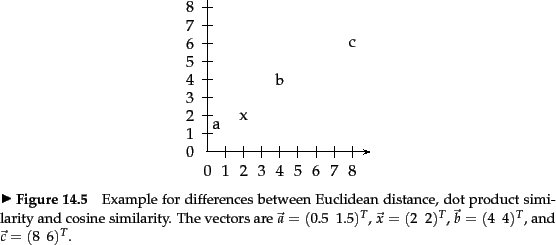Exercises.

• In Figure 14.13 , which of the three vectors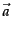,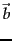, and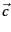is (i) most similar to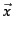according to dot product similarity, (ii) most similar toaccording to cosine similarity, (iii) closest toaccording to Euclidean distance?

• Download Reuters-21578 and train and test Rocchio and kNN classifiers for the classes acquisitions, corn, crude, earn, grain, interest, money-fx, ship, trade, and wheat. Use the ModApte split. You may want to use one of a number of software packages that implement Rocchio classification and kNN classification, for example, the Bow toolkit (McCallum, 1996).

• Download 20 Newgroups (page 8.2 ) and train and test Rocchio and kNN classifiers for its 20 classes.

• Show that the decision boundaries in Rocchio classification are, as in kNN, given by the Voronoi tessellation.

• Computing the distance between a dense centroid and a sparse vector is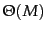for a naive implementation that iterates over all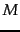dimensions. Based on the equality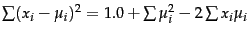and assuming that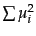has been precomputed, write down an algorithm that is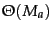instead, where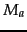is the number of distinct terms in the test document.

• Prove that the region of the plane consisting of all points with the same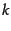nearest neighbors is a convex polygon.

• Design an algorithm that performs an efficient 1NN search in 1 dimension (where efficiency is with respect to the number of documents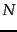). What is the time complexity of the algorithm?

• Design an algorithm that performs an efficient 1NN search in 2 dimensions with at most polynomial (in) preprocessing time.

• Can one design an exact efficient algorithm for 1NN for very largealong the ideas you used to solve the last exercise?

• Show that Equation 145 defines a hyperplane with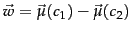and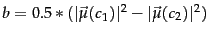.

• We can easily construct non-separable data sets in high dimensions by embedding a non-separable set like the one shown in Figure 14.14 . Consider embedding Figure 14.14 in 3D and then perturbing the 4 points slightly (i.e., moving them a small distance in a random direction). Why would you expect the resulting configuration to be linearly separable? How likely is then a non-separable set of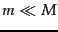points in-dimensional space?

• Assuming two classes, show that the percentage of non-separable assignments of the vertices of a hypercube decreases with dimensionalityfor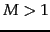. For example, for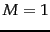the proportion of non-separable assignments is 0, for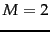, it is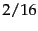. One of the two non-separable cases foris shown in Figure 14.14 , the other is its mirror image. Solve the exercise either analytically or by simulation.

• Although we point out the similarities of Naive Bayes with linear vector space classifiers, it does not make sense to represent count vectors (the document representations in NB) in a continuous vector space. There is however a formalization of NB that is analogous to Rocchio. Show that NB assigns a document to the class (represented as a parameter vector) whose Kullback-Leibler (KL) divergence (Section 12.4 , page 12.4 ) to the document (represented as a count vector as in Section 13.4.1 (page), normalized to sum to 1) is smallest.Next: Support vector machines and Up: Vector space classification Previous: References and further reading   Contents   Index